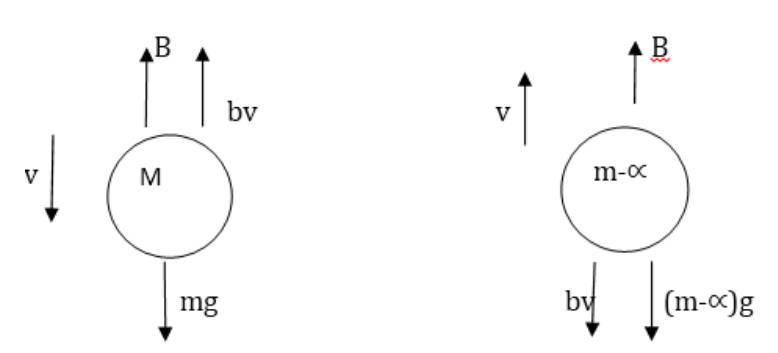# Solve the following :`
Question:

The force of buoyancy exerted by the atmosphere on a balloon is $B$ in the upward direction and remains constant. The force of air resistance on the balloon acts opposite to the direction of velocity and is proportional to it. The balloon carries a mass $M$ and is found to fall down near the earth's surface with a constant velocity v. How much mass should be removed from the balloon so that it may rise with a constant velocity v?Solution:

$\mathrm{B}+\mathrm{bv}=\mathrm{mg}$

$B=b v+(m-\propto) g$

$\mathrm{Bv}$ is constant

$M g-B=B-\left(M^{\propto}\right) g$

$2 \mathrm{Mg}=2 \mathrm{~B}+{ }^{\propto} \mathrm{g}$

$\propto_{=2}\left(M m-\frac{B}{g}\right)$